# CS 109A/AC 209A/STAT 121A Data Science: Homework 4¶

Harvard University
Fall 2016
Instructors: W. Pan, P. Protopapas, K. Rader
Due Date: Wednesday, October 5th, 2016 at 11:59pm

Download the IPython notebook as well as the data file from Vocareum and complete locally.

To submit your assignment, in Vocareum, upload (using the 'Upload' button on your Jupyter Dashboard) your solution to Vocareum as a single notebook with following file name format:

last_first_CourseNumber_HW4.ipynb

where CourseNumber is the course in which you're enrolled (CS 109a, Stats 121a, AC 209a). Submit your assignment in Vocareum using the 'Submit' button.

Avoid editing your file in Vocareum after uploading. If you need to make a change in a solution. Delete your old solution file from Vocareum and upload a new solution. Click submit only ONCE after verifying that you have uploaded the correct file. The assignment will CLOSE after you click the submit button.

Problems on homework assignments are equally weighted. The Challenge Question is required for AC 209A students and optional for all others. Student who complete the Challenge Problem as optional extra credit will receive +0.5% towards your final grade for each correct solution.

Import libraries

In :
import numpy as np
import pandas as pd
from sklearn.linear_model import LinearRegression as Lin_Reg
from sklearn.linear_model import Ridge as Ridge_Reg
from sklearn.linear_model import Lasso as Lasso_Reg
from statsmodels.regression.linear_model import OLS
import sklearn.preprocessing as Preprocessing
from sklearn.cross_validation import train_test_split
from sklearn.cross_validation import KFold
from sklearn.cross_validation import cross_val_score
import itertools as it
import matplotlib
import matplotlib.pyplot as plt
import matplotlib.cm as cmx
import matplotlib.colors as colors
import scipy as sp
from itertools import combinations
%matplotlib inline


# I'm a title¶

## Problem 0: Basic Information¶

[Hagmann, Tim]

[CS 109a]

### Part (c): Who did you work with?¶

-

All data sets can be found in the datasets folder and are in comma separated value (CSV) format

## Problem 1: Variable selection and regularization¶

The data set for this problem is provided in dataset_1.txt and contains 10 predictors and a response variable.

### Part (a): Analyze correlation among predictors¶

• By visually inspecting the data set, do find that some of the predictors are correlated amongst themselves?
• Compute the cofficient of correlation between each pair of predictors, and visualize the matrix of correlation coefficients using a heat map. Do the predictors fall naturally into groups based on the correlation values?
• If you were asked to select a minimal subset of predictors based on the correlation information in order to build a good regression model, how many predictors will you pick, and which ones will you choose?
In :
# Load data

# Split predictors and response
x = data[:, :-1]
y = data[:, -1]

df = pd.DataFrame(data)

Out:
0 1 2 3 4 5 6 7 8 9 10
0 0.959357 0.959357 0.959357 0.343727 0.524083 0.537768 0.435598 0.831999 0.153247 0.005016 0.289394
1 0.616969 0.616969 0.616969 0.287376 0.513844 0.497775 0.452732 0.914609 0.367390 0.444473 -0.277574
2 0.995941 0.995941 0.995941 0.107294 0.097106 0.146751 0.136414 0.635926 0.535209 0.899457 -0.513097
3 0.821732 0.821732 0.821732 0.202558 0.329504 0.359471 0.281453 0.106263 0.479327 0.256271 -0.182353
4 0.302423 0.302423 0.302423 0.184564 0.270263 0.293385 0.263866 0.378630 0.740241 0.468589 -0.625117
In :
# Compute matrix of correlation coefficients
corr_matrix = np.corrcoef(x.T)
pd.DataFrame(corr_matrix)

Out:
0 1 2 3 4 5 6 7 8 9
0 1.000000 1.000000 1.000000 -0.189945 -0.232439 -0.215623 -0.228322 -0.047643 -0.061022 0.050977
1 1.000000 1.000000 1.000000 -0.189945 -0.232439 -0.215623 -0.228322 -0.047643 -0.061022 0.050977
2 1.000000 1.000000 1.000000 -0.189945 -0.232439 -0.215623 -0.228322 -0.047643 -0.061022 0.050977
3 -0.189945 -0.189945 -0.189945 1.000000 0.974279 0.964344 0.969540 0.058516 0.108294 -0.010416
4 -0.232439 -0.232439 -0.232439 0.974279 1.000000 0.982785 0.982227 0.080342 0.126004 -0.012399
5 -0.215623 -0.215623 -0.215623 0.964344 0.982785 1.000000 0.976308 0.079547 0.123368 -0.021029
6 -0.228322 -0.228322 -0.228322 0.969540 0.982227 0.976308 1.000000 0.074004 0.112241 -0.022023
7 -0.047643 -0.047643 -0.047643 0.058516 0.080342 0.079547 0.074004 1.000000 -0.133182 0.052151
8 -0.061022 -0.061022 -0.061022 0.108294 0.126004 0.123368 0.112241 -0.133182 1.000000 0.006923
9 0.050977 0.050977 0.050977 -0.010416 -0.012399 -0.021029 -0.022023 0.052151 0.006923 1.000000
In :
# Display heat map
fig, ax = plt.subplots(1, 1, figsize=(6, 6))

ax.pcolor(corr_matrix)

ax.set_title('Heatmap of correlation matrix')

plt.show()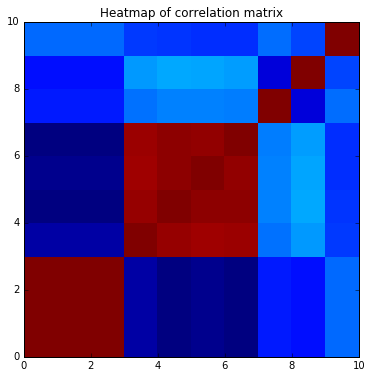** Comment: **
pc It also shows that there are natural groups amongst them. There is a correlated group among the variables 0 to 2 and 3 to 6.

When building a good regression model with a subset i would pick on of the variables 0 to 2, one 3 to 6 and 7, 8 and 9, i.e., 5 variables.

### Part (b): Selecting minimal subset of predictors¶

• Apply the variable selection methods discussed in class to choose a minimal subset of predictors that yield high prediction accuracy:

• Exhaustive search

• Step-wise forward selection or Step-wise backward selection

In each method, use the Bayesian Information Criterion (BIC) to choose the subset size.

• Do the chosen subsets match the ones you picked using the correlation matrix you had visualized in Part (a)?

Note: You may use the statsmodels's OLS module to fit a linear regression model and evaluate BIC. You may not use library functions that implement variable selection.

In :
### Best Subset Selection
min_bic = 1e10 # set some initial large value for min BIC score
best_subset = [] # best subset of predictors

# Create all possible subsets of the set of 10 predictors
predictor_set = set(range(10)) # predictor set = {0, 1, 2, 3, 4, 5, 6, 7, 8, 9}

# Repeat for every possible size of subset
for size_k in range(10):
# Create all possible subsets of size 'size',
# using the 'combination' function from the 'itertools' library
subsets_of_size_k = it.combinations(predictor_set, size_k + 1)

max_r_squared = -1e10 # set some initial small value for max R^2 score
best_k_subset = [] # best subset of predictors of size k

# Iterate over all subsets of our predictor set
for predictor_subset in subsets_of_size_k:
# Use only a subset of predictors in the training data
x_subset = x[:, predictor_subset]

# Fit and evaluate R^2
model = OLS(y, x_subset)
results = model.fit()
r_squared = results.rsquared

# Update max R^2 and best predictor subset of size k
# If current predictor subset has a higher R^2 score than that of the best subset
# we've found so far, remember the current predictor subset as the best!
if(r_squared > max_r_squared):
max_r_squared = r_squared
best_k_subset = predictor_subset[:]

# Use only the best subset of size k for the predictors
x_subset = x[:, best_k_subset]

# Fit and evaluate BIC of the best subset of size k
model = OLS(y, x_subset)
results = model.fit()
bic = results.bic

# Update minimum BIC and best predictor subset
# If current predictor has a lower BIC score than that of the best subset
# we've found so far, remember the current predictor as the best!
if(bic < min_bic):
min_bic = bic
best_subset = best_k_subset[:]

print('Best subset by exhaustive search:')
print sorted(best_subset)

Best subset by exhaustive search:
[0, 5, 7, 8, 9]

In :
### Step-wise Forward Selection
d = x.shape # total no. of predictors

# Keep track of current set of chosen predictors, and the remaining set of predictors
current_predictors = []
remaining_predictors = range(d)

# Set some initial large value for min BIC score for all possible subsets
global_min_bic = 1e10

# Keep track of the best subset of predictors
best_subset = []

# Iterate over all possible subset sizes, 0 predictors to d predictors
for size in range(d):
max_r_squared = -1e10 # set some initial small value for max R^2
best_predictor = -1 # set some throwaway initial number for the best predictor to add
bic_with_best_predictor = 1e10 # set some initial large value for BIC score

# Iterate over all remaining predictors to find best predictor to add
for i in remaining_predictors:
# Make copy of current set of predictors
temp = current_predictors[:]
temp.append(i)

# Use only a subset of predictors in the training data
x_subset = x[:, temp]

# Fit and evaluate R^2
model = OLS(y, x_subset)
results = model.fit()
r_squared = results.rsquared

# Check if we get a higher R^2 value than than current max R^2, if so, update
if(r_squared > max_r_squared):
max_r_squared = r_squared
best_predictor = i
bic_with_best_predictor = results.bic

# Remove best predictor from remaining list, and add best predictor to current list
remaining_predictors.remove(best_predictor)
current_predictors.append(best_predictor)

# Check if BIC for with the predictor we just added is lower than
# the global minimum across all subset of predictors
if(bic_with_best_predictor < global_min_bic):
best_subset = current_predictors[:]
global_min_bic = bic_with_best_predictor

print 'Step-wise forward subset selection:'
print sorted(best_subset) # add 1 as indices start from 0

Step-wise forward subset selection:
[0, 5, 7, 8, 9]

In :
###  Step-wise Backward Selection
d = x.shape # total no. of predictors

# Keep track of current set of chosen predictors
current_predictors = range(d)

# First, fit and evaluate BIC using all 'd' number of predictors
model = OLS(y, x)
results = model.fit()
bic_all = results.bic

# Set the minimum BIC score, initially, to the BIC score using all 'd' predictors
global_min_bic = bic_all
# Keep track of the best subset of predictors
best_subset = []

# Iterate over all possible subset sizes, d predictors to 1 predictor
for size in range(d - 1, 1, -1): # stop before 0 to avoid choosing an empty set of predictors
max_r_squared = -1e10 # set some initial small value for max R^2
worst_predictor = -1 # set some throwaway initial number for the worst predictor to remove
bic_without_worst_predictor = 1e10 # set some initial large value for min BIC score

# Iterate over current set of predictors (for potential elimination)
for i in current_predictors:
# Create copy of current predictors, and remove predictor 'i'
temp = current_predictors[:]
temp.remove(i)

# Use only a subset of predictors in the training data
x_subset = x[:, temp]

# Fit and evaluate R^2
model = OLS(y, x_subset)
results = model.fit()
r_squared = results.rsquared

# Check if we get a higher R^2 value than than current max R^2, if so, update
if(r_squared > max_r_squared):
max_r_squared = r_squared
worst_predictor = i
bic_without_worst_predictor = results.bic

# Remove worst predictor from current set of predictors
current_predictors.remove(worst_predictor)

# Check if BIC for the predictor we just removed is lower than
# the global minimum across all subset of predictors
if(bic_without_worst_predictor < global_min_bic):
best_subset = current_predictors[:]
global_min_bic = bic_without_worst_predictor

print 'Step-wise backward subset selection:'
print sorted(best_subset)

Step-wise backward subset selection:
[2, 5, 7, 8, 9]


** Comment: **
The subset selection shows that similar variables as the ones picked by me are chosen, i.e., [2, 5, 7, 8, 9] or [0, 5, 7, 8, 9].

### Part (c): Apply Lasso and Ridge regression¶

• Apply Lasso regression with regularization parameter $\lambda = 0.01$ and fit a regression model.

• Identify the predictors that are assigned non-zero coefficients. Do these correspond to the correlation matrix in Part (a)?
• Apply Ridge regression with regularization parameter $\lambda = 0.01$ and fit a regression model.

• Is there a difference between the model parameters you obtain different and those obtained from Lasso regression? If so, explain why.

• Identify the predictors that are assigned non-zero coefficients. Do these correspond to the correlation matrix in Part (a)?

• Is there anything peculiar that you observe about the coefficients Ridge regression assigns to the first three predictors? Do you observe the same with Lasso regression? Give an explanation for your observation.

Note: You may use the statsmodels or sklearn to perform Lasso and Ridge regression.

In :
# Lasso regression
reg = Lasso_Reg(alpha = 0.01)
reg.fit(x, y)
coefficients = reg.coef_

print 'Lasso:'
print 'Coefficients:', coefficients
print  'Predictors with non-zero coefficients:', [i for i, item in enumerate(coefficients) if abs(item) > 0]

Lasso:
Coefficients: [ 0.02717417  0.          0.         -0.         -0.02532806 -0.         -0.
0.04397321 -0.40612185 -0.22260474]
Predictors with non-zero coefficients: [0, 4, 7, 8, 9]


** Comment: **
The predictors correspond with the correlation matrix, i.e., [0, 4, 7, 8, 9]

In :
# Ridge regression: Fit and evaluate
reg = Ridge_Reg(alpha = 0.01)
x[:,1] = x[:,0]
x[:,2] = x[:,0]
reg.fit(x, y)
coefficients = reg.coef_

print 'Ridge:'
print 'Coefficients:', coefficients
print 'Predictors with non-zero coefficients:', [i for i, item in enumerate(coefficients) if abs(item) > 0]

Ridge:
Coefficients: [ 0.04353543  0.04353543  0.04353543  0.55217415 -0.19706852 -0.61421737
0.30484213  0.18742866 -0.50083242 -0.35908145]
Predictors with non-zero coefficients: [0, 1, 2, 3, 4, 5, 6, 7, 8, 9]


** Comment: **
As is to be expected, the ridge regression selects all variables, i.e., [0, 1, 2, 3, 4, 5, 6, 7, 8, 9]. That means it doesn't correspond with the correlation matrix.

The ridge regression assigns exactly the same value to the variables 0 to 2. This is because there is a prefect i.e., 1:1 correlation between those variables. The lasso regression doesn't show the same behavior, as there is only on of those variables selected.

## Problem 2: Cross-validation and Bootstrapping¶

In this problem, you will work with an expanded version of the automobile pricing data set you analyzed in Homework 3. The data set is contained dataset_2.txt, with 26 attribues (i.e. predictors) for each automobile and corresponding prices.

### Part(a): Encode categorical attributes and fill missing values¶

Identify the categorical attributes in the data. Replace their values with the one-hot binary encoding. You may do this using the get_dummies() function in pandas. If you do this task correctly, you should get a total of 69 predictors after the encoding.

In :
## Load data

# Split predictors and response
y = data.iloc[:, -1]
x = data.iloc[:, :-1]

## Transformations
# Transform symboling into categorical variable
x['symboling'] = x['symboling'].astype(object)

# Get categorical values
categorical_bolean = (x.dtypes == "object").values
x_numeric = x.loc[:, ~categorical_bolean]
x_categorical = x.loc[:, categorical_bolean]
x_dummies = pd.get_dummies(x_categorical)

## Scale the numerical x variables
x_numeric = x_numeric / x_numeric.std(axis=0)

## Bind data together
x = pd.concat([x_numeric, x_dummies], axis=1)
print x.shape

(205, 69)


### Part (b): Apply regular linear regression¶

• Split the data set into train and test sets, with the first 25% of the data for training and the remaining for testing.
• Use regular linear regression to fit a model to the training set and evaluate the R^2 score of the fitted model on both the training and test sets. What do you observe about these values?
• You had seen in class that the R^2 value of a least-squares fit to a data set would lie between 0 and 1. Is this true for the test R^2 values reported above? If not, give a reason for why this is the case.
• Is there a need for regularization while fitting a linear model to this data set?

Note: You may use the statsmodels or sklearn to fit a linear regression model and evaluate the fits.

In :
## Split the dataset
np.random.seed(856) # Set seed
x_train, x_test = train_test_split(x, test_size = 0.25)

## Split the variables
y_train = y.iloc[x_train.index.values]
y_test = y.iloc[x_test.index.values]
x_train = x.iloc[x_train.index.values, :]
x_test = x.iloc[x_test.index.values, :]

## Transform to numpy
y_train = y_train.values
y_test = y_test.values
x_train = x_train.values
x_test = x_test.values

In :
# First, let's do plain OLS
reg = Lin_Reg()
reg.fit(x_train, y_train)

train_r_squared_plain = reg.score(x_train, y_train)
test_r_squared_plain = reg.score(x_test, y_test)

print 'Plain Regression: R^2 score on training set', train_r_squared_plain
print 'Plain Regression: R^2 score on test set', test_r_squared_plain

Plain Regression: R^2 score on training set 0.990955195397
Plain Regression: R^2 score on test set 0.958059163439


** Comment: **
The R^2 values of the model are very high, i.e., 99% in the training an 95% in the test set. That is a near perfect fit. However, as is to be expected, the training set overfits the data a bit. Which can be noticed by the smaller test R^2. As it should be, both values lie between 0 and 1.

In this case of the linear regression it is not necessary to regularize the data.

### Part (c): Apply Ridge regression¶

• Apply Ridge regression on the training set for different values of the regularization parameter $\lambda$ in the range $\{10^{-7}, 10^{-6}, \ldots, 10^7\}$. Evaluate the R^2 score for the models you obtain on both the train and test sets. Plot both values as a function of $\lambda$.
• Explain the relationship between the regularization parameter and the training and test R^2 scores.
• How does the best test R^2 value obtained using Ridge regression compare with that of plain linear regression? Explain.

Note: You may use the statsmodels or sklearn to fit a ridge regression model and evaluate the fits.

In :
# Ridge regression: Fit and evaluate
reg = Ridge_Reg(alpha = 1.0)
reg.fit(x_train, y_train)
coefficients = reg.coef_

predictors = [i for i, item in enumerate(coefficients) if abs(item) > 0]

print 'Ridge:'
print 'Coefficients:', coefficients
print 'Selected predictors:',predictors
print 'Ridge Regression: R^2 score on training set', reg.score(x_train,y_train)
print 'Ridge Regression: R^2 score on test set', reg.score(x_test,y_test)

Ridge:
Coefficients: [  992.92148619   553.97771546   444.35687821  -828.94054753  -525.36834772
2211.93526343   459.23600141  1160.12392818  4443.04676286 -1125.6272756
-1718.78978862   315.37876754   344.79954543  -758.59928852  -924.65800278
-36.75443242   457.93905479  1014.82987063  -630.91902486   119.56253464
395.33569594  2884.31772629    77.27639028 -1644.3110965     13.51645961
-704.2174629   3183.18495578 -1863.20872571 -1458.68909567  -836.10191045
-667.66261737 -1441.07097977  2494.76231488   567.89881667  1449.1084432
-2179.99901015  -893.98887089  -364.09680086   987.94576763    71.98632035
-71.98632035  -905.22298642   905.22298642   181.93047182  -181.93047182
2266.97626872   459.87867544  -736.78619848  -871.37469    -1118.69405569
37.65252076    17.84309103   -55.49561178 -2998.30613842  2998.30613842
77.68045447  -667.66261737  1229.61288637   818.30712827 -1457.93785174
2794.18813447  -659.19799568  -128.44268015 -2006.54745864    13.51645961
494.53946992    71.98632035    79.19678992  -659.2390398 ]
Selected predictors: [0, 1, 2, 3, 4, 5, 6, 7, 8, 9, 10, 11, 12, 13, 14, 15, 16, 17, 18, 19, 20, 21, 22, 23, 24, 25, 26, 27, 28, 29, 30, 31, 32, 33, 34, 35, 36, 37, 38, 39, 40, 41, 42, 43, 44, 45, 46, 47, 48, 49, 50, 51, 52, 53, 54, 55, 56, 57, 58, 59, 60, 61, 62, 63, 64, 65, 66, 67, 68]
Ridge Regression: R^2 score on training set 0.981590182809
Ridge Regression: R^2 score on test set 0.97329430963

In :
# Run for different values of lambda
lambda_min = -7
lambda_max = 7

num_lambdas = 15
num_predictors = x.shape

lambdas= np.linspace(lambda_min,lambda_max, num_lambdas)

train_r_squared = np.zeros(num_lambdas)
test_r_squared = np.zeros(num_lambdas)

coeff_a = np.zeros((num_lambdas, num_predictors))

In :
for ind, i in enumerate(lambdas):
# Fit ridge regression on train set
reg = Ridge_Reg(alpha = 10**i)
reg.fit(x_train, y_train)

coeff_a[ind,:] = reg.coef_
# Evaluate train & test performance
train_r_squared[ind] = reg.score(x_train, y_train)
test_r_squared[ind] = reg.score(x_test, y_test)

In :
# Plotting
plt.figure(figsize=(18, 8))
plt.plot(train_r_squared, 'bo-', label=r'$R^2$ Training set', color="darkblue", alpha=0.6, linewidth=3)
plt.plot(test_r_squared, 'bo-', label=r'$R^2$ Test set', color="darkred", alpha=0.6, linewidth=3)
plt.xlabel('Lamda value'); plt.ylabel(r'$R^2$')
plt.xlim(0, 14)
plt.title(r'Evaluate ridge regression $R^2$ with different lamdas')
plt.legend(loc='best')
plt.grid()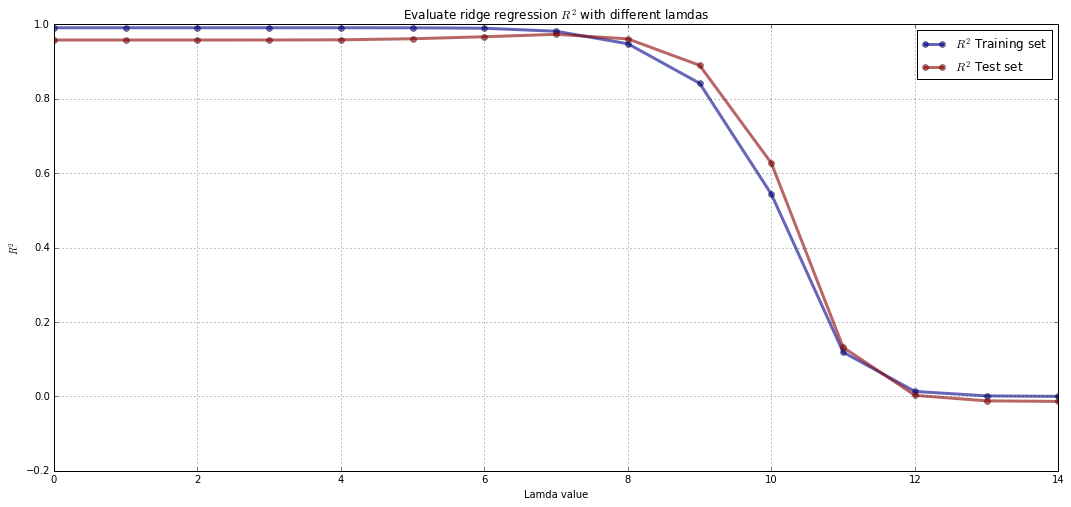** Comment: **
The best R^2 in the test set is reached when the lamda value is 0, i.e., when a standard linear regression is performed. That means that with this particular data set the variables shouldn't be scaled back. A lamda of zero means a OLS is preformerd while when the lamda goes to infinity the coefficients go to zero.

### Part (d): Tune regularization parameter using cross-validation and bootstrapping¶

• Evaluate the performance of the Ridge regression for different regularization parameters $\lambda$ using 5-fold cross validation or bootstrapping on the training set.

• Plot the cross-validation (CV) or bootstrapping R^2 score as a function of $\lambda$.

• How closely does the CV score or bootstrapping score match the R^2 score on the test set? Does the model with lowest CV score or bootstrapping score correspond to the one with maximum R^2 on the test set?

• Does the model chosen by CV or bootstrapping perform better than plain linear regression?

Note: You may use the statsmodels or sklearn to fit a linear regression model and evaluate the fits. You may also use kFold from sklearn.cross_validation.

In :
# Run for different values of lambda
lambda_min = -7
lambda_max = 7

num_lambdas = 15
num_predictors = x.shape

lambdas= np.linspace(lambda_min,lambda_max, num_lambdas)

train_r_squared = np.zeros(num_lambdas)
test_r_squared = np.zeros(num_lambdas)

In :
kfold = KFold(n=len(x), n_folds=5, random_state=123)

for ind, i in enumerate(lambdas):
# Fit ridge regression on train set
reg = Ridge_Reg(alpha = 10**i)
reg.fit(x_train, y_train)
results = cross_val_score(reg, x, y, cv=kfold, scoring="r2")

# Evaluate train & test performance
train_r_squared[ind] = results.mean()
test_r_squared[ind] = reg.score(x_test, y_test)

In :
# Plotting
plt.figure(figsize=(18, 8))
plt.plot(train_r_squared, 'bo-', label=r'$R^2$ Training set', color="darkblue", alpha=0.6, linewidth=3)
plt.plot(test_r_squared, 'bo-', label=r'$R^2$ Test set', color="darkred", alpha=0.6, linewidth=3)
plt.xlabel('Lamda value'); plt.ylabel(r'$R^2$')
plt.xlim(0, 14)
plt.title(r'Evaluate 5-fold cv with different lamdas')
plt.legend(loc='best')
plt.grid()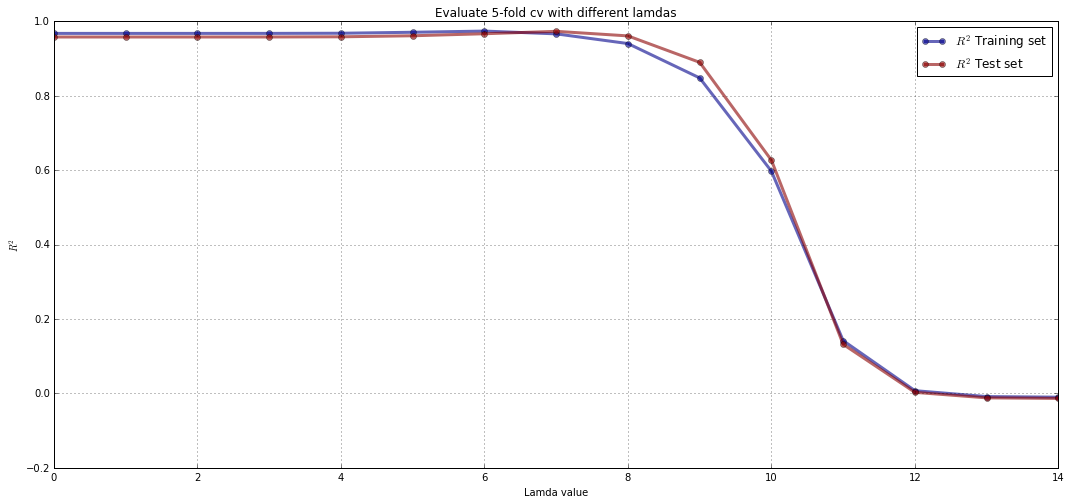** Comment: **
The $R^2$ for the test set from the cross validation follows a similar shape to the $R^2$ score on the test set from the other regressions.

The maximum $R^2$ from cross validation is achieved for $\lambda = 0$ and from ridge regression prediction on the test set, the maximum value of $R^2$ is also achieved with $\lambda = 0$. That mean both the CV and the ridge regression select the same model as the OLS.

The lowest MSE is achieved for $\lambda = 0$ which corresponds to the model selected by the R^2 measure.

As all the models tend to go to lamda = 0 they perform the same as the OLS regression.

## Problem 3: Ridge regression via ordinary least-squares regression¶

We present an approach to implement Ridge regression using oridinary least-squares regression. Given a matrix of responses $\mathbf{X} \in \mathbb{R}^{n\times p}$ and response vector $\mathbf{y} \in \mathbb{R}^{n}$, one can implement Ridge regression with regularization parameter $\lambda$ as follows:

• Augment the matrix of predictors $\mathbf{X}$ with $p$ new rows containing the scaled identity matrix $\sqrt{\lambda}\mathbf{I} \in \mathbb{R}^{p \times p}$, i.e. $$\overline{\mathbf{X}} \,=\, \begin{bmatrix} X_{11} & \ldots & X_{1p}\\ \vdots & \ddots & \vdots\\ X_{n1} & \ldots & X_{np}\\ \sqrt{\lambda} & \ldots & 0\\ \vdots & \ddots & \vdots\\ 0 & \ldots & \sqrt{\lambda} \end{bmatrix} \,\in\, \mathbb{R}^{(n+p)\times p} .$$
• Augment the response vector $\mathbf{y}$ with a column of $p$ zeros, i.e. $$\overline{\mathbf{y}} \,=\, \begin{bmatrix} y_{1}\\ \vdots\\ y_{n}\\ 0\\ \vdots\\ 0 \end{bmatrix} \,\in\, \mathbb{R}^{n+p}.$$
• Apply ordinary least-squares regression on the augmented data set $(\overline{\mathbf{X}}, \overline{\mathbf{y}})$.

### Part (a): Show the proposed approach implements Ridge regression¶

Show that the approach proposed above implements Ridge regression with parameter $\lambda$.

** Solution: **
The ridge regression takes the following form: $$L(\beta) = (Y-X\beta)^T(Y-X\beta) + \lambda\beta^T\beta$$

The normal equations for ridge regression is: $$(X^T\,X + \lambda I)\beta = X^T Y$$

so $\beta$ is solved by using the following matrix inverse: $$\beta = (X^T\,X + \lambda I)^{-1} X^T Y$$

X and Y can be enhanced so that the normal equation has the same form as for ordinary least squeres. That means including the $\lambda\beta^2$ into an $\overline{\mathbf{X}}$ matrix and $\overline{\mathbf{y}}$ vector. As $\lambda >0$ the square root $\sqrt{\lambda}$ will be positive so the matrix X (an n×p matrix) can be augemented by appending the p x p matrix $\sqrt{\lambda}I$, and the vector Y can be extended by appending a column of p zeros. The product $(\overline{\mathbf{y}}-\overline{\mathbf{X}}\beta)^T(\overline{\mathbf{y}}-\overline{\mathbf{X}}\beta)$ adds an additional p terms to the objective function of ordinary least squares of the form:

$$(0 - \sqrt{\lambda}\beta_i)^2 = \lambda\beta^T\beta$$ which equals to the additional term in the function for the ridge regression.

That means that the normal equation for $\beta$ using the ridge regression can be rewritten as $$(Y-X\beta)^T(Y-X\beta) + \lambda\beta^2 = (\overline{\mathbf{y}}-\overline{\mathbf{X}}\beta)^T(\overline{\mathbf{y}}-\overline{\mathbf{X}}\beta)$$

From the right the normal equation can then be rewritten as: $${\overline{\mathbf{X}}}^T\,\overline{\mathbf{X}}\beta = {\overline{\mathbf{X}}}^T \overline{\mathbf{y}}$$

which then can be solved using OLS.

The function for ridge regression takes the form: $$F(\beta) = (Y-X\beta)^T(Y-X\beta) + \lambda\beta^T\beta$$

The function for OLS regression with $(\overline{\mathbf{X}}, \overline{\mathbf{y}})$ takes the form: $$F(\beta)= (\overline{\mathbf{y}} - \overline{\mathbf{X}})^T(\overline{\mathbf{y}} - \overline{\mathbf{X}})$$

$\overline{\mathbf{y}}^T\overline{\mathbf{y}}=y^Ty$, as the bottom p terms of $\overline{\mathbf{y}}$ are all zero.

$\overline{\mathbf{y}}^T\overline{\mathbf{X}}=y^TX$, as the bottom p terms of $\overline{\mathbf{y}}$ are all zero.

$\overline{\mathbf{X}}^T\overline{\mathbf{X}}=\Big( X^T \ \sqrt{\lambda}I_p \Big) \begin{pmatrix}X \sqrt{\lambda}I_p\end{pmatrix}$.

That means, $$L_{OLS}({\beta}) = (\overline{\mathbf{y}}-\overline{\mathbf{X}}\beta)^T(\overline{\mathbf{y}} - \overline{\mathbf{X}}\beta)$$

$$= \overline{\mathbf{y}}^T\overline{\mathbf{y}} -2\overline{\mathbf{y}}^T\overline{\mathbf{X}} \beta + \beta^T \overline{\mathbf{X}}^T\overline{\mathbf{X}} \beta$$

$$= y^Ty -2y^TX + \beta^T (X^T X + \lambda I_p) \beta$$ $$= y^Ty -2y^TX + \beta^T(X^T X)\beta + \lambda \beta^T\beta$$ $$= (y-X\beta)^T(y-X\beta) + \lambda\beta^T\beta$$ $$= L_{RIDGE}(\beta)$$

That shows that the approach proposed implements Ridge regression with parameter $\lambda$.

### Part (b): Debug our implementation of ridge regression¶

You're a grader for CS109A, the following is an implemention of Ridge regression (via the above approach) submitted by a student. The dataset is dataset_3.txt. The regression model is fitted to a training set, and the R^2 scores of the fitted model on the training and test sets are plotted as a function of the regularization parameter. Grade this solution according to the following rubric (each category is equally weighted):

• correctness

• interpretation (if applicable)

• code/algorithm design

• presentation

In addition to providing an holistic grade (between 0 to 5), provide a corrected version of this code that is submission quality.

In :
# Fit
def ridge(x_train, y_train, reg_param):
n=np.shape(x_train)
x_train=np.concatenate((x_train,reg_param*np.identity(n)),axis=1)
y_train_=np.zeros((n+np.shape(x_train),1))
for c in range(n):
y_train_[c]= y_train[c]
import sklearn
model = sklearn.linear_model.LinearRegression()
model.fit(x_train,y_train.reshape(-1,1))
return model

# Score
def score(m,x_test,y_test, reg_param):
n=np.shape(x_train)
x_test=np.concatenate((x_test,reg_param*np.identity(n)),axis=1)
y_test_=np.zeros((n+np.shape(x_test),1))
for c in range(n):
y_test_[c]= y_test[c]
return m.score(x_test,y_test.reshape(-1,1))

n = data.shape
n = int(np.round(n*0.5))
x_train = data[0:n,0:100]
y_train = data[0:n,100]
x_test = data[n:2*n,0:100]
y_test = data[n:2*n,100]

# Params
a=np.zeros(5)
for i in range(-2,2):
a[i+2]=10**i

# Iterate
rstr =np.zeros(5)
rsts =np.zeros(5)
for j in range(0,5):
m =ridge(x_train,y_train,a[i])
rstr[j]=score(m,x_train,y_train,a[j])
rsts[i]=score(m,x_test,y_test,a[i])

# Plot
plt.plot(a,rstr)
plt.plot(a,rsts)

Out:
[<matplotlib.lines.Line2D at 0x10f887f0>]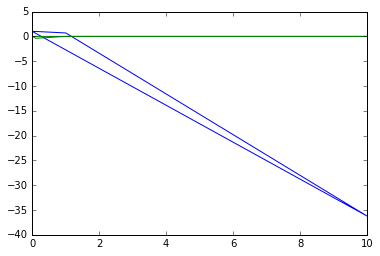** Corrected version: **

In :
# Load packages
from sklearn.linear_model import LinearRegression as Lin_Reg

In :
# Functions:
# Fit
def ridge(x_train, y_train, reg_param):
n, p = np.shape(x_train) # x is an n x p matrix
x_train = np.concatenate((x_train, np.sqrt(10.0**reg_param)*np.identity(p)), axis=0)
y_train = np.concatenate((y_train, np.zeros(p)), axis=0)
model = Lin_Reg()
model.fit(x_train, y_train)
return model

# Score
def score(m, x_test,y_test, reg_param):
n, p = np.shape(x_train) # x is an n x p matrix
x_test = np.concatenate((x_test, np.sqrt(10.0**reg_param)*np.identity(p)), axis=0)
y_test = np.concatenate((y_test, np.zeros(p)), axis=0)
return m.score(x_test, y_test)

In :
# Load

# Extract out x and y
x_df = data[:, 0:-1]
y_df = data[:, -1]

n = data.shape
n_train = int(np.round(n*0.5))

# shuffle data and split into test and train sets
indices = np.random.permutation(range(n))
train_indices = indices[0:n_train]
test_indices = indices[n_train:]

x_standardised = x_df

x_train = x_standardised[train_indices,:]
y_train = y_df[train_indices]
x_test = x_standardised[test_indices,:]
y_test = y_df[test_indices]

In :
# Generate an array of lambda values
lambdas=np.linspace(-2,2, 100)
num_lambdas = len(lambdas)

# Initialise empty arrays for storing r2 values
r2_train =np.zeros(num_lambdas)
r2_test =np.zeros(num_lambdas)

In :
# Iterate over the values of lambda
for i in range(num_lambdas):
# fit a ridge regression model on the training set
model = ridge(x_train,y_train,lambdas[i])
# predict on the test
r2_train[i]=score(model, x_train,y_train,lambdas[i])
r2_test[i]=score(model, x_test,y_test, lambdas[i])

In :
# Ploting
fig, ax = plt.subplots(1, 1, figsize=(18, 8))
ax.semilogx(10.0**lambdas, (r2_train), c='b', label='Ridge: Train')
ax.semilogx(10.0**lambdas, (r2_test), c='r', label='Ridge: Test')

ax.set_xlabel('Regularization parameter $\lambda$')
ax.set_ylabel(r'$R^2$ score')
ax.legend(loc = 'best')
plt.grid()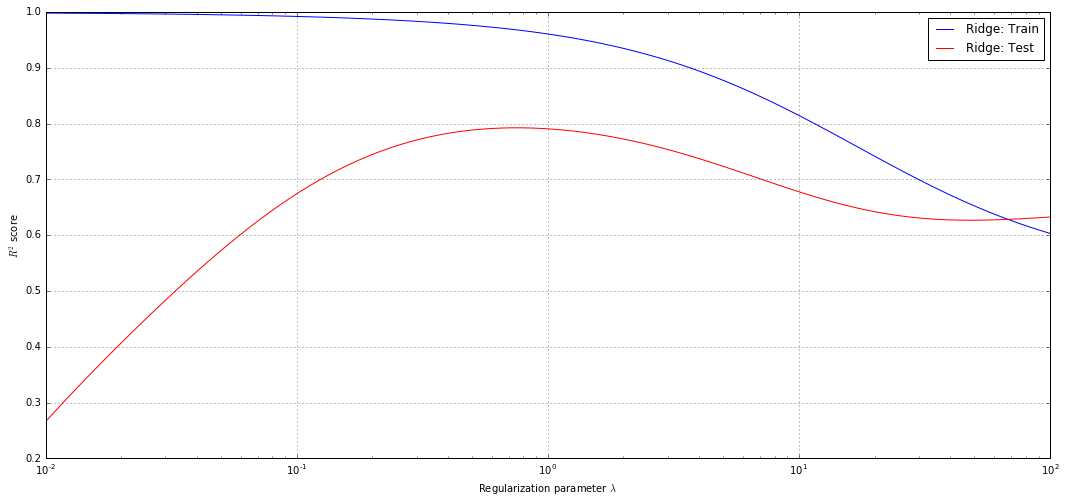** Comment: **
The best R^2 in the test set is reached with 0.79259, i.e., when the lamda value is slightly smaller than 10'0.

Correctness
-The student made a couple of errors in the code.

Interpretation (if applicable)

Code/algorithm design
-The student used poor labelling for the variables
-The student didn't really comment in the code

Presentation
-No axes labels in the plots and no legend

## Challenge Problem: Predicting Outcome of a Fund-raising Campaign¶

You are provided a data set containing details of mail sent to 95,412 potential donors for a fund-raising campaign of a not-for-profit organization. This data set also contains the amount donated by each donor. The task is to build a model that can estimate the amount that a donor would donate using his/her attributes. The data is contained in the file dataset_4.txt. Each row contains 376 attributes for a donor, followed by the donation amount.

Note: For additional information about the attributes used, please look up the file dataset_4_description.txt. This files also contains details of attributes that have been omitted from the data set.

### Part (a): Fit regression model¶

Build a suitable model to predict the donation amount. How good is your model?